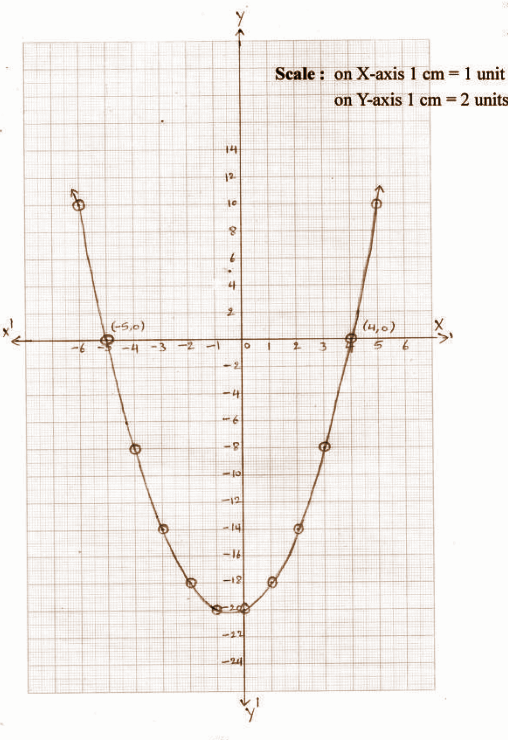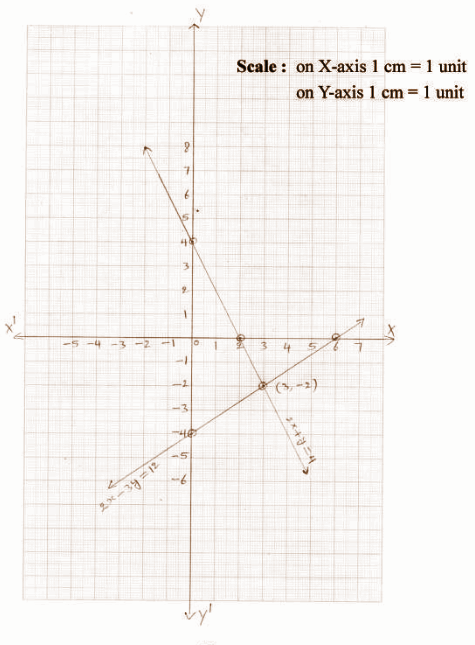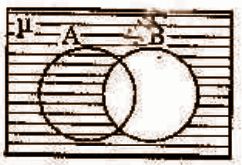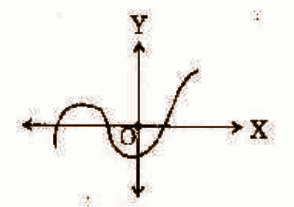PRINTABLE FOR KIDS

XII (12) HSC

XI (11) FYJC
X (10) SSC

### Andhra Pradesh SSC Class 10th Maths Question Paper 1 With Solution 2019 QUESTION PAPER CODE 15E(A)

SECTION – I

(4 * 1 = 4)

Question 1: Write A = {2, 4, 8, 16} in set-builder form.

Solution:

A = {2n / n ∈ N, and n < 5}

Question 2: Find the value of log5 √625.

Solution:

log5 √625

= log5 25

log52

= 2 log5 5

= 2 * 1

= 2

Question 3: The larger of two supplementary angles exceeds the smaller by 58o, then find the angles.

Solution:

Let the required supplementary angles be x and y.

x + y = 180o —- (1)

The larger angle exceeds the smaller by 58o.

x – y = 58o —- (2)

Solve (1) and (2),

2x = 238

x = 238 / 2

x = 119o

y = 61o

Question 4: Find the curved surface area of the cylinder, whose radius is 7cm and height is 10cm.

Solution:

Radius if the cylinder (r) = 7cm

Height of the cylinder (h) = 10cm

The curved surface area of the cylinder = 2𝛑rh

= 2 * (22 / 7) * (7) * (10)

= 440 cm2

SECTION – II

(5 * 2 = 10)

Question 5: Rohan’s mother is 26 years older than him. The product of their ages after 3 years will be 360. Then write the required quadratic equation to find Rohan’s present age.

Solution:

Let Rohan’s present age be x years.

His mother’s age at present is (x + 26) years.

After 3 years, Rohan’s age = (x + 3) years

After 3 years, his mother’s age = (x + 26) + 3 = (x + 29) years

The product of their ages = (x + 3) (x + 29)

= x * x + x * 29 + 3 * x + 3 * 29

= x2 + 29x + 3x + 87

= x2 + 32x + 87

By the sum, the product of their ages is 360

x2 + 32x + 87 = 360

x2 + 32x + 87 – 360 = 0

x2 + 32x – 273 = 0 is the required quadratic equation.

Question 6: Find the zeroes of the quadratic polynomial x2 – x – 30 and verify the relation between the zeroes and its coefficients.

Solution:

Given the polynomial x2 – x – 30,

To find the zeros, x2 – x – 30 = 0 [say]

= x2 – 6x + 5x – 30

= x (x – 6) + 5 (x – 6)

= (x + 5) (x – 6)

x = -5 and x = 6

Sum of the zeroes = 6 + (-5)

= 1

= (-1) / 1

= – (coefficient of x) / (coefficient of x2)

Product of the zeroes = 6 (-5)

= -30

= (-30 / 1)

= (constant term) / (coefficient of x2)

Question 7: A joker’s cap is in the form of a right circular cone, whose base radius is 7cm and height is 24cm. Find the area of the sheet required to make 10 such caps.

Solution:

Base radius of the conical cap (r) = 7cm

Height (h) = 24cm

Slant height (l) = √r2 + h2

= √72 + 242

= √49 + 576

= √625

= 25 cm

Area of sheet required to make a cap = Lateral surface area of the cap

= 𝛑rl

= (22 / 7) * 7 * 25

= 550 sq.cm

Area of sheet required to 10 such caps

= 10 * 550

= 5500 sq.cm

Question 8: Find the HCF of 1260 and 1440 by using Euclid’s division lemma.

Solution:

The given numbers are 1260 and 1440.

1440 = 1260 * 1 + 180

1260 = 180 * 7 + 0

HCF of 1440 and 1260 is 180.

Question 9: If the sum of the first 15 terms of an AP is 675 and its first term is 10, then find 25th term.

Solution:

First-term of an AP = a = 10

Let the common difference be d.

Sum of the first 15 terms is S15 = 675

(15 / 2) = [2a + 14d] = 675

[2 * 10] + 14d = (675 * 2) / 15

14d = 90 – 20 = 70

d = 70 / 14

d = 5

25th term of an AP is a25 = a + 24d

= 10 + 24 * 5

= 10 + 120

= 130

SECTION – III

(4 * 4 = 16)

Question 10:

[a] Show that 2 + 5√3 is irrational.

OR

[b] Check whether -321 is a term of the AP 22, 5, 8, 1…..

Solution:

[a] Let us assume the contrary that 2 + 5√3 is rational that is coprime can be found for ‘a’ and ‘b’ and b ≠ 0 such that

2 + 5√3 = (a / b)

5√3 = (a / b) – 2

√3 = (a / 5b) – (2 / 5)

Since (a / 5b) and (2 / 5) ∈ Q, (a / 5b) – (2 / 5) ∈ Q.

So, √3 is rational.

But this contradicts the fact that √3 is irrational.

So, our assumption that 2 + 5√3 is rational is wrong.

So, 2 + 5√3 is irrational.

[b] From the given AP, 22, 15, 8, 1 …..

a = 22, d = -7

nth term of an AP = an = a + (n – 1)d

In this AP, let the nth term be -321

a + (n – 1)d = -321

22 + (n – 1) (-7) = -321

(n – 1) (-7) = -343

n – 1 = (-343) / (-7)

n = 49 + 1

n = 50

Hence, -321 will be the 50th term in the given AP.

Question 11:

[a] In a class test, the sum of Moulika’s marks in mathematics and English is 30. If she got 2 marks more in mathematics and 3 marks less in English, the product of her marks would have been 210. Find her marks in the two subjects.

OR

[b] An oil drum is in the shape of the cylinder, whose diameter is 2m and height is 7m. The painter charges Rs. 5 per m2 to paint the drum. Find the total charges to be paid to the painter for 10 drums.

Solution:

[a] Given that the sum of Moulika’s marks in Mathematics and English is 30.

Let the marks of Moulika in Mathematics be x and that of in English be 30 – x.

If she got 2 marks more in mathematics then marks in maths = x + 2

And she got 3 marks less in English then the marks in English = 30 – x – 3 = 27 – x

Product of these two = (x + 2) (27 – x) = 210

x– 25x + 156 = 0

(x – 12) (x – 13) = 0

x = 12, 13

Case (i) If x = 12, the marks of Moulika in

Mathematics = 12

English = 30 – 12 = 18

Case (ii) If x = 13, the marks of Moulika in

Mathematics = 13

English = 30 – 13 = 17

[b] The diameter of the oil drum which is in the shape of cylinder = d = 2m

The radius of the drum = r = d / 2 = 2 / 2 = 1m

Height = h = 7cm

Total surface area of the drum which is in the shape of cylinder = 2𝛑r (r + h)

= 2 * (22 / 7) * (1) * (1 + 7)

= 2 * (22 / 7) * 8

= 50.28 sq.m

Charges to paint the drum per sq.m = Rs. 5

The total cost of painting 10 such type of drums = 50.28 * 5 * 10 = Rs. 2514

Question 12:

(i) [a] If A = {x : x is a natural number less than is 6}.

B = {x : x is a prime number which is a divisor of 60}.

C = {x : x is an odd natural number less than 10}.

D = {x : x is an even natural number which is a divisor of 48}.

Then write the roster form for all the above sets and find

[a] A ⋃ B

[b] B ⋂ C

[c] A – D

[d] D – B

OR

(ii) 6 pencils and 4 notebooks together cost Rs. 90 whereas 8 pencils and 3 notebooks together cost Rs. 85. Find the cost of one pencil and that of one notebook.

Solution:

(i) [a] A = {1, 2, 3, 4, 5}

B = {2, 3, 5}

C = {1, 3, 5, 7, 9}

D = {2, 4, 6, 8, 12, 14, 16, 24, 48}

[a] A ⋃ B = {1, 2, 3, 4, 5} ⋃ {2, 3, 5} = {1, 2, 3, 4, 5}

[b] B ⋂ C = {2, 3, 5} ⋂ {1, 3, 5, 7, 9} = {3, 5}

[c] A – D = {1, 2, 3, 4, 5} – {2, 4, 6, 8, 12, 14, 16, 24, 48} = {1, 3, 5}

[d] D – B = {2, 4, 6, 8, 12, 14, 16, 24, 48} – {2, 3, 5} = {4, 6, 8, 12, 16, 24, 48}

(ii) Let the cost of one pencil be Rs. x.

Cost of one notebook = Rs. y.

The total cost of 6 pencils and 4 notebooks = Rs. 90

6x + 4y = 90 —- (1)

The total cost of 8 pencils and 3 notebooks = Rs. 85

8x + 3y = 85 —- (2)

On solving the above two equations, x = 5, y = 15.

The cost of one pencil = Rs. 5

The cost of one notebook = Rs. 15

Question 13:

[a] Find the zeroes of the quadratic polynomial p(x) = x2 + x – 20 using the graph.

OR

[b] Solve the following pair of linear equations graphically.

2x + y + 4 and 2x – 3y = 12.

Solution:

[a] Let y = x2 + x – 20[b]SECTION – IV

(20 * 0.5 = 10)

Question 14: If n(A) = 8, n (B) = 3, n (A ⋂ B) = 2, then n (A ⋃ B) = ______

(A) 5 (B) 7 (C) 9 (D) 13

Question 15: The discriminant of 6x2 – 5x + 1 = 0 is ______

(A) 1 (B) 2 (C) 6 (D) (-5 / 6)

Question 16: Sum of the zeroes of the polynomial x2 + 5x + 6 = 0 is

(A) 5 (B) -5 (C) 6 (D) (5 / 6)

Question 17: Which of the following is not irrational?

(A) √2 (B) √3 (C) √4 (D) √5

Question 18: One root of the equation x – (3 / x) = 2 is _____

(A) 1 (B) 2 (C) 3 (D) 4

Question 19: If 4, a, 9 are in GP, then a = ____

(A) 6 (B) ±6 (C) 7 (D) ±7

Question 20: If the total surface area of a cube is 96cm2, then its volume is _____

(A) 32cm3 (B) 64 cm3 (C) 128cm3 (D) 256 cm3

Question 21: log 10 0.001 = _____

(A) 2 (B) 3 (C) -2 (D) -3

Question 22: Match the following.

If a, b, c are the zeroes of a cubic polynomial ax3 + bx2 + cx + d = 0, then

[i] a + b + c [a] (-d / a)

[ii] ab + bc + ca [b] (c / a)

[iii] abc [c] (-b / a)

(A) [i] – c, [ii] – b, [iii] – a

(B) [i] – a, [ii] – b, [iii] – c

(C) [i] – b, [ii] – a, [iii] – c

(D) [i] – c, [ii] – a, [iii] – b

Question 23: The next term in AP √3, √12, √27, ……

(A) √32 (B) √36 (C) √42 (D) √48

Question 24: The shaded region in the figure shows(A) A – B (B) B – A (C) μ – B (D) A ⋃ B

Question 25: 5x – 3 represents ______ polynomial.

(A) Linear (B) Quadratic (C) Cubic (D) Fourth degree

Question 26: The common difference in AP log2 2, log2 4, log2 8 …… is

(A) 1 (B) 2 (C) 3 (D) 4

Question 27: The sum of the first ‘n’ odd natural numbers is ______

(A) n (B) n2 (C) n (n + 1) (D) n (n + 1) / 2

Question 28: The quadratic polynomial, whose zeroes are √2 and √-2 is ____

(A) x2 – 2 (B) x2 + 2 (C) x2 + √2 (D) x – 2

Question 29: The number of zeroes of the polynomial in the graph is _____(A) 0 (B) 1 (C) 2 (D) 3

Question 30: The line 2x – 3y = 8 intersects x-axis at

(A) (2, -3) (B) (0, -3) (C) (2, 0) (D) (4, 0)

Question 31: The volume of the cone, whose radius is 3cm and height is 8cm, is _____ cm3.

(A) 6𝛑 (B) 12𝛑 (C) 18𝛑 (D) 24𝛑

Question 32: If 6x + 2y + 9 = 0 and kx + y – 7 = 0 has no solution, then k = ______

(A) 3 (B) 2 (C) -3 (D) -2

Question 33: If the equation x2 + 5x + k = 0 has real and distinct roots, then ____

(A) k = 6 (B) k < 6.25 (C) k > 6 (D) k > 25

## PDF FILE TO YOUR EMAIL IMMEDIATELY PURCHASE NOTES & PAPER SOLUTION. @ Rs. 50/- each (GST extra)

SUBJECTS

HINDI ENTIRE PAPER SOLUTION

MARATHI PAPER SOLUTION
SSC MATHS I PAPER SOLUTION
SSC MATHS II PAPER SOLUTION
SSC SCIENCE I PAPER SOLUTION
SSC SCIENCE II PAPER SOLUTION
SSC ENGLISH PAPER SOLUTION
SSC & HSC ENGLISH WRITING SKILL
HSC ACCOUNTS NOTES
HSC OCM NOTES
HSC ECONOMICS NOTES
HSC SECRETARIAL PRACTICE NOTES

2019 Board Paper Solution

HSC ENGLISH SET A 2019 21st February, 2019

HSC ENGLISH SET B 2019 21st February, 2019

HSC ENGLISH SET C 2019 21st February, 2019

HSC ENGLISH SET D 2019 21st February, 2019

SECRETARIAL PRACTICE (S.P) 2019 25th February, 2019

HSC XII PHYSICS 2019 25th February, 2019

CHEMISTRY XII HSC SOLUTION 27th, February, 2019

OCM PAPER SOLUTION 2019 27th, February, 2019

HSC MATHS PAPER SOLUTION COMMERCE, 2nd March, 2019

HSC MATHS PAPER SOLUTION SCIENCE 2nd, March, 2019

SSC ENGLISH STD 10 5TH MARCH, 2019.

HSC XII ACCOUNTS 2019 6th March, 2019

HSC XII BIOLOGY 2019 6TH March, 2019

HSC XII ECONOMICS 9Th March 2019

SSC Maths I March 2019 Solution 10th Standard11th, March, 2019

SSC MATHS II MARCH 2019 SOLUTION 10TH STD.13th March, 2019

SSC SCIENCE I MARCH 2019 SOLUTION 10TH STD. 15th March, 2019.

SSC SCIENCE II MARCH 2019 SOLUTION 10TH STD. 18th March, 2019.

SSC SOCIAL SCIENCE I MARCH 2019 SOLUTION20th March, 2019

SSC SOCIAL SCIENCE II MARCH 2019 SOLUTION, 22nd March, 2019

XII CBSE - BOARD - MARCH - 2019 ENGLISH - QP + SOLUTIONS, 2nd March, 2019

HSC Maharashtra Board Papers 2020

(Std 12th English Medium)

HSC ECONOMICS MARCH 2020

HSC OCM MARCH 2020

HSC ACCOUNTS MARCH 2020

HSC S.P. MARCH 2020

HSC ENGLISH MARCH 2020

HSC HINDI MARCH 2020

HSC MARATHI MARCH 2020

HSC MATHS MARCH 2020

SSC Maharashtra Board Papers 2020

(Std 10th English Medium)

English MARCH 2020

HindI MARCH 2020

Hindi (Composite) MARCH 2020

Marathi MARCH 2020

Mathematics (Paper 1) MARCH 2020

Mathematics (Paper 2) MARCH 2020

Sanskrit MARCH 2020

Important-formula

THANKS